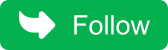## Thursday, May 5, 2022

### Question 78 : Write an algorithm to implement insertion sort?

Insertion sort  works by comparing values at index with all its prior elements.We place value at the index where there are no lesser value to the elements. So when you reach last element,we get a sorted array.

Lets see how it works:
Lets say you have array as `{100,20,15,30,5,75}`

1. Compare 20 with 100,as 20 is less than 100, our array will become `{100,100,15,30,5,75}`. We have reached to 0th index, we will place 20 to 0th index `{20,100,15,30,5,75}`
2. Compare 15 with 100, as it is less,our array will become `{20,100,100,30,5,75}`. Now compare 20 with 15, it is again less, our array will become `{20,20,100,30,5,75}`. As we have reached start of array again. Place 15 at 0th index.our array will become `{15,20,100,30,5,75}`
3. Compare 30 with 100, as it is less,our array will become `{15,20,100,100,5,75}`.Now compare 30 with 20 again, it is more, so we will stop here, As we reached at index where 30 is greater than 15 and 20. so we will put 30 at this index. our array will become `{15,20,30,100,5,75}`
4. Compare 5 with 100, as it is less, our array will become `{15,20,30,100,100,75}`. Now compare 30 with 5 again,it is less,our array will become `{15,20,30,30,100,75}`. Similarly 20 with 5, it is less,our array will become `{15,20,20,30,100,75}`.Compare 15 with 5, it is less,our array will become `{15,15,20,30,100,75}`.As we have reached start of array again. Place 5 at 0th index.our array will become `{5,15,20,30,100,75}`
5. Compare 75 with 100, it is less,so our array will become `{5,15,20,30,100,100}`.Now compare 30 with 75 again, it is more, so we will stop here, As we reached at index where 75 is greater than 5,15,20 and 30. so we will put 75 at this index. our array will become `{5,15,20,30,75,100}`
6. Finally we got sorted array.

## Insertion sort implementation

I have printed intermediate steps to understand it better:

``````Public class InsertionSortMain {

public static void main(String args[])
{
int  arr[]={100,20,15,30,5,75};
insertionSort(arr);

}

public static int[] insertionSort(int arr[])
{
for (int i = 1; i < arr.length; i++)
{
int valueToSort = arr[i];
int j;
// If we get smaller value than valueToSort then , we stop at that index.
for ( j = i; j > 0 && arr[j - 1] > valueToSort; j--) {
arr[j] = arr[j - 1];
}

// We will put valueToSort at that index
arr[j] = valueToSort;
System.out.print("Iteration "+(i)+": ");
printArray(arr);
}

return arr;
}
public static void printArray(int arr[])
{
for (int i = 0; i <arr.length; i++) {
System.out.print(arr[i]+" ");
}
System.out.println();
}
}``````
When you run above program, you will get the following output:
``````Iteration 1: 20 100 15 30 5 75
Iteration 2: 15 20 100 30 5 75
Iteration 3: 15 20 30 100 5 75
Iteration 4: 5 15 20 30 100 75
Iteration 5: 5 15 20 30 75 100``````

We are iterating from first element to last element.You may notice that each iteration places current element at its right place where all its prior element are less than current element.

## Time Complexity

Best case: `O(n)`
Average case: `O(n^2)`
Worst case: `O(n^2)`

Don't miss the next article!

Be the first to be notified when a new article or Kubernetes experiment is   published.